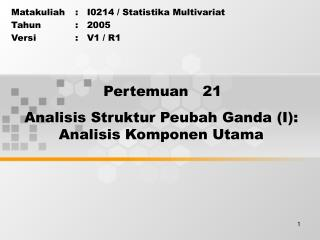DownloadDownload PresentationPertemuan 21

# Pertemuan 21

Télécharger la présentation## Pertemuan 21

- - - - - - - - - - - - - - - - - - - - - - - - - - - E N D - - - - - - - - - - - - - - - - - - - - - - - - - - -
##### Presentation Transcript

1. Pertemuan 21 Analisis Struktur Peubah Ganda (I):Analisis Komponen Utama Matakuliah : I0214 / Statistika Multivariat Tahun : 2005 Versi : V1 / R1

2. Learning Outcomes Pada akhir pertemuan ini, diharapkan mahasiswa akan mampu : • Mahasiswa dapat menganalisis struktur peubah ganda  C4 • Mahasiswa dapat menggunakan analisis komponen utama  C3

3. Outline Materi • Konsep dasar analisis komponen utama • Analisis komponen utama

4. <<ISI>> • Overview of PCA • One of the major objectives in exploratory data analysis of • multivariate data is dimension reduction. • 􀀔To screen data for obvious outliers. • 􀀔To select low-dimensional projections of the data for graphing. • 􀀔To search for “structure” in the data. • The primary statistical tool to accomplish this is through the creation • of Principal Components. • A principal component is defined as a linear combination or • projection of optimally-weighted observed variables. • (In appearance, this linear function is similar to a multiple regression • equation except that there is no intercept term).

5. <<ISI>>

6. <<ISI>>

7. <<ISI>>

8. <<ISI>>

9. <<ISI>>

10. <<ISI>>

11. <<ISI>>

12. <<ISI>>

13. <<ISI>>

14. <<ISI>>

15. <<ISI>>

16. <<ISI>>

17. <<ISI>>

18. <<ISI>>

19. <<ISI>>

20. <<ISI>>

21. <<ISI>>

22. <<ISI>> PCA Issues Sample Size No less than 50 observations, better to have 100. Rule of thumb is to have at least 20 observations per variable. Measurement Scales Theory assumes continuous variables. If all variables are binary use correspondence analysis. Original or Standardized Variables Principle component analysis can be performed on standardized variables (i.e. assessing the correlation matrix), or unstandardized values (I.e. using the covariance matrix). Standardized scores aid comparisons among different variables, especially when those variables have quite different variances. The difference in variances can be very important in the definition of components. Number or Variables PCA can be performed on any number of variables. With large numbers of variables there is a much higher chance that some of the components will have zero or very small eigenvalues indicating exact or near collinearity. Variables that do not weigh highly in the more significant components may be dropped and components recomputed. Removed variables can be analyzed in their own separate analysis.

23. <<ISI>> Number of Components to Plot How many components to plot will depend on the relative values of the eigenvalues and the analysts criteria as to how much of the total variation must be explained. Typical criteria are described below: • Latent Root Criterion Plot combinations of components having eigenvalues >1. Use fewer factors if the number of variables is less than 50 and more if the number of variables greater than 50 • Percentage of Variance Criterion Consider components important until the fraction of explained variance exceeds some pre-specified level, say 95% in the natural sciences or 60% in the social sciences, or when the last component added adds less than 5% • Scree Test Criterion Examination of the Scree Plot to identify the number of components where the curve first begins to straighten out

24. <<ISI>>

25. <<ISI>> • PCA Summary • Principal components analysis is a powerful and useful multivariate technique for reducing the dimensions of data sets with large numbers of continuous variables • Because PCA utilizes only linear combinations, the ordering of the distances between points in p-dimensional space is not changed • Can be used to develop measures capable of representing a number of observed variables (The first couple of components) • There is a high degree of subjectivity in deciding on the number of components to examine and interpretation of those components • PCA is usually applied to the correlation matrix associated with a set of variables (R-mode) or observations (Q-mode). It may also be applied to the covariance matrix of observations that have been centered but not scaled

26. << CLOSING>> • Sampai dengan saat ini Anda telah mempelajari kosep dasar analisis komponen utama • Untuk dapat lebih memahami konsep dasar analisis komponen utama tersebut, cobalah Anda pelajari materi penunjang, website/internet dan mengerjakan latihan